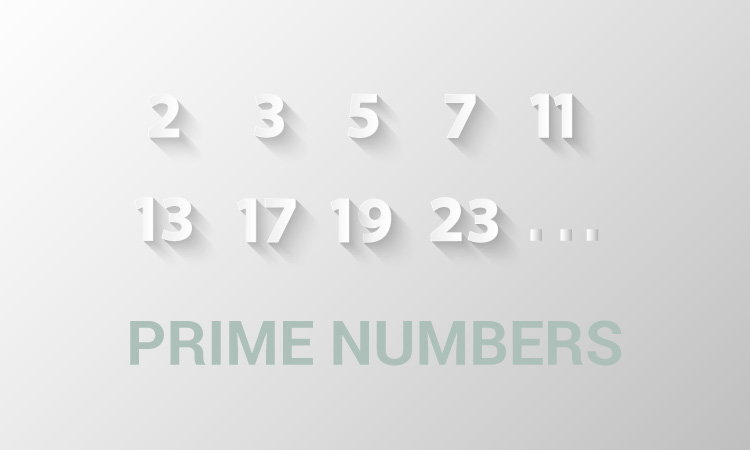# C Program to Check Whether a Number is Prime or Not

In this example, you will learn to check whether an integer entered by the user is a prime number or not.To understand this example, you should have the knowledge of the following C programming topics:

A prime number is a positive integer that is divisible only by `1` and itself. For example: 2, 3, 5, 7, 11, 13, 17

## Program to Check Prime Number

``````#include <stdio.h>
int main() {
int n, i, flag = 0;
printf("Enter a positive integer: ");
scanf("%d", &n);

for (i = 2; i < n / 2; ++i) {

// condition for non-prime
if (n % i == 0) {
flag = 1;
break;
}
}

if (n == 1) {
printf("1 is neither prime nor composite.");
}
else {
if (flag == 0)
printf("%d is a prime number.", n);
else
printf("%d is not a prime number.", n);
}

return 0;
}
``````

Output

```Enter a positive integer: 29
29 is a prime number.
```

In the program, a for loop is iterated from `i = 2` to `i < n/2`.

In each iteration, whether n is perfectly divisible by i is checked using:

``````if (n % i == 0) {
�
}
``````

If n is perfectly divisible by i, n is not a prime number. In this case, flag is set to 1, and the loop is terminated using the `break` statement.

After the loop, if n is a prime number, flag will still be 0. However, if n is a non-prime number, flag will be 1.

Visit this page to learn how you can print all the prime numbers between two intervals.